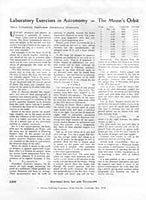# Lab Exercises in Astronomy: The Moon's Orbit

• \$1.65
Unit price per

In this classic lab exercise, students measure photographs of the Moon to calculate the orbital elements for the Moon's orbit. The exercise is designed for a 90-minute class, but can be shortened as needed.

As the Moon revolves around the Earth in its noncircular orbit, its apparent size undergoes a change of about 10 percent. In this exercise, devised by John C. Duncan at Wellesley College, the student measures the Moon’s diameter as accurately as possible on 12 photographs taken during a month. Each measure is multiplied by a factor to permit plotting the distance it represents against the Moon’s geocentric longitude for the date. On the resulting polar plot, the 12 points can be joined by a smooth curve (nearly an ellipse) that shows the shape of the Moon’s orbit. The student finds perigee and apogee, draws the line of apsides, works out the law of areas, finds the orbital eccentricity, and draws the line of nodes from latitudes tabulated in the experiment.

LE001